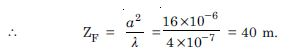# NCERT Solutions for Class 12 Physics Chapter 4 - Wave Optics

The NCERT Solutions on Aasoka are available for free to help students get an understanding of the topics related to the chapter “Wave Optics”. The NCERT Solutions for Class 12th will help students to get ready for their board exams along with competitive exams such as JEE and NEET. This chapter is thoroughly covered by the subject matter experts as per the latest syllabus. They have made sure to include the crucial concept or topic questions so that students do not miss out on anything important that might come in the exams.

With the help of the “Wave Optics” chapter of Physics Class 12th, students get knowledge about Huygen’s principle, Brewster’s Law, angular width expression, proof of laws of refraction and reflection utilizing Huygen’s principle, coherent sources, the width of central maximum, sustained interference of light, Young’s double-slit experiment, wavefront, refraction and reflection of plane wave at a plane surface, etc.

##### Question 1:

Monochromatic light of wavelength 589 nm is incident from air on a water surface.What are the wavelength, frequency and speed of (a) reflected and (b) refracted light ? Refractive index of water is 1.33.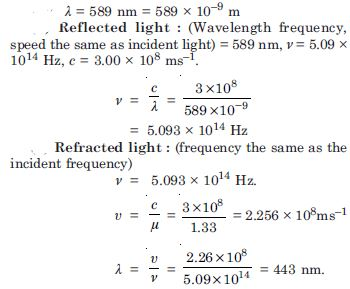##### Question 2:

What is the shape of the wavefront in each of the following cases :

Light diverging from a point source.
Light emerging out of a convex lens when a point source is placed at its focus.
The portion of the wavefront of light from a distant star intercepted by the earth.

It is spherical wavefront.
It is plane wavefront.
Plane wavefront (a small area on the surface of a large sphere is nearly planar.)

##### Question 3:

The refractive index of glass is 1.5. What is the speed of light in glass ? (Speed of light in vacuum is 3.0 × 108 ms–1).
Is the speed of light in glass independent of the colour of light ? If not, which of the two colours red and violet, travels slower in a glass prism ?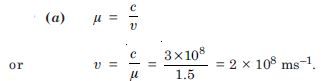No. The refractive index, and hence the speed of light in a medium, depends on wavelength, [When no particular wavelength or colour of light is specified, we may take the given refractive index to refer to yellow colour]. Now we know violet colour deviates more than red in glass i.e. $\mathrm{\mu }$V > $\mathrm{\mu }$R. Therefore the violet component of white light travels slower than the red component.

##### Question 4:

In a Young’s double slit experiment, the slits are separated by 0.28 mm and the screen is placed 1.4 m away. The distance between the central bright fringe and the fourth bright fringe is measured to be 1.2 cm. Determine the wavelength of light used in the experiment.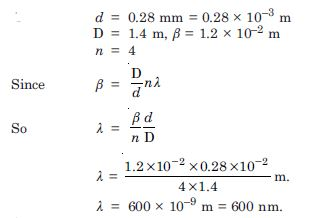##### Question 5:

In Young’s double slit experiment using monochromatic light of wavelength $\mathrm{\lambda }$, the intensity of light at a point on the screen where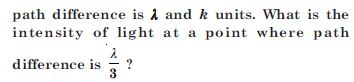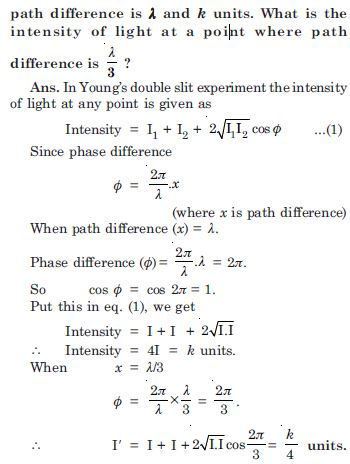##### Question 6:

A beam of light consisting of two wavelengths 650 nm and 520 nm, is used to obtain interference fringes in a Young’s double slit experiment.

Find the distance of the third bright fringe on the screen from the central maximum for wavelength 650 nm.

What is the least distance from the central maximum where the bright fringes due to both the wavelengths coincide ? The distance between the slits is 2 mm and the distance between the plane of slits and screen is 120 cm.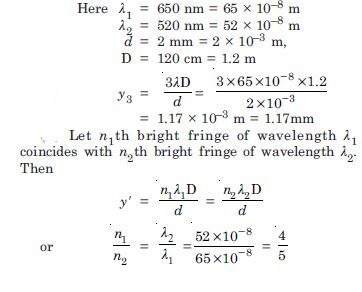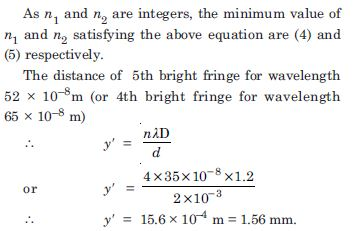##### Question 7:

In a double slit experiment the angular width of a fringe is found to be 0.2$°$ on a screen placed 1 m away. The wavelength of light used is 600 nm. What will be the angular width of the fringe if the entire experimnental apparatus is immersed in water ? Take refractive index of water to be 4/3.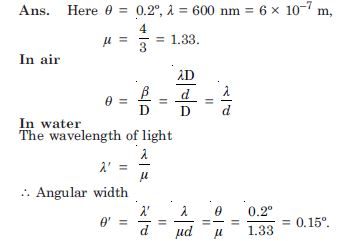##### Question 8:

What is the Brewster angle for air to glass transition ? (refractive index of glass = 1.5).

Given
$\mathrm{\mu }$ = 1.5, $\mathrm{\theta }$ = ?
Since $\mathrm{\mu }$ = tan $\mathrm{\theta }$
$\therefore$ tan $\mathrm{\theta }$ = 1.5
or $\mathrm{\theta }$ = tan–1 1.5 = 56.3$°$.

##### Question 9:

Light of wavelength 5000 Å falls on a reflecting surface. What are the wavelength and frequency of the reflected light ? For what angle of incidence is the reflected ray normal to the incident ray ?

Given $\mathrm{\lambda }$ = 5000 Å = 5 × 10–7 m
The wavelength and frequency of reflected light remains the same.
$\therefore$ Wavelength of reflected light,
$\mathrm{\lambda }$ = 5000 Å
Frequency of reflected light,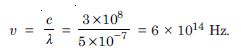The reflected ray is normal to incident if angle of incidence i = 45$°$.

##### Question 10:

Estimate the distance for which ray optics is good approximation for an aperture of 4 mm and wavelength 400 nm.

$\mathrm{\lambda }$ = 400 nm = 4 × 10 –7 m
$\therefore$ The minimum distance a beam of light has totravel before its deviation from straight line path becomes significant is called Fresnel distance ZF